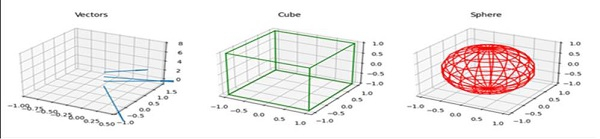# Plotting a 3d cube, a sphere and a vector in Matplotlib

Get fig from plt.figure() and create three different axes using add_subplot, where projection=3d.

Set up the figure title using ax.set_title("name of the figure"). Use the method ax.quiver to plot vector projection, plot3D for cube, and plot_wireframe for sphere after using sin and cos.

## Steps

• Create a new figure, or activate an existing figure.

• To draw vectors, get a 2D array.

• Get a zipped object.

• Add an ~.axes.Axes to the figure as part of a subplot arrangement, with 3d projection, where nrows = 1, ncols = 3 and index = 1.

• Plot a 3D field of arrows.

• Set xlim, ylim and zlim.

• Set the title of the axis (at index 1).

• Add an ~.axes.Axes to the figure as part of a subplot arrangement, with 3d projection, where nrows = 1, ncols = 3 and index = 2.

• Create a surface plot using plot3D(), where the surface and edge are being passed with color green.

• Set the title of the axis (at index 2). i.e., “Cube”.

• Add an ~.axes.Axes to the figure as part of a subplot arrangement, with 3d projection, where nrows = 1, ncols = 3 and index = 3.

• To make the sphere get sin and cos curves together at the same position.

• Set the title of the axis (at index 3), i.e., “Sphere”.

• To show the plot, use plt.show() method.

## Example

import matplotlib.pyplot as plt
import numpy as np
from itertools import product, combinations

fig = plt.figure()

# draw vector
soa = np.array([[0, 0, 1, 1, -2, 0], [0, 0, 2, 1, 1, 0],
[0, 0, 3, 2, 1, 0], [0, 0, 4, 0.5, 0.7, 0]])

X, Y, Z, U, V, W = zip(*soa)
ax.quiver(X, Y, Z, U, V, W)
ax.set_xlim([-1, 0.5])
ax.set_ylim([-1, 1.5])
ax.set_zlim([-1, 8])
ax.set_title("Vectors")

# draw cube
r = [-1, 1]
for s, e in combinations(np.array(list(product(r, r, r))), 2):
if np.sum(np.abs(s-e)) == r-r:
ax.plot3D(*zip(s, e), color="green")
ax.set_title("Cube")

# draw sphere
u, v = np.mgrid[0:2*np.pi:20j, 0:np.pi:10j]
x = np.cos(u)*np.sin(v)
y = np.sin(u)*np.sin(v)
z = np.cos(v)
ax.plot_wireframe(x, y, z, color="red")
ax.set_title("Sphere")

plt.show()

## Output